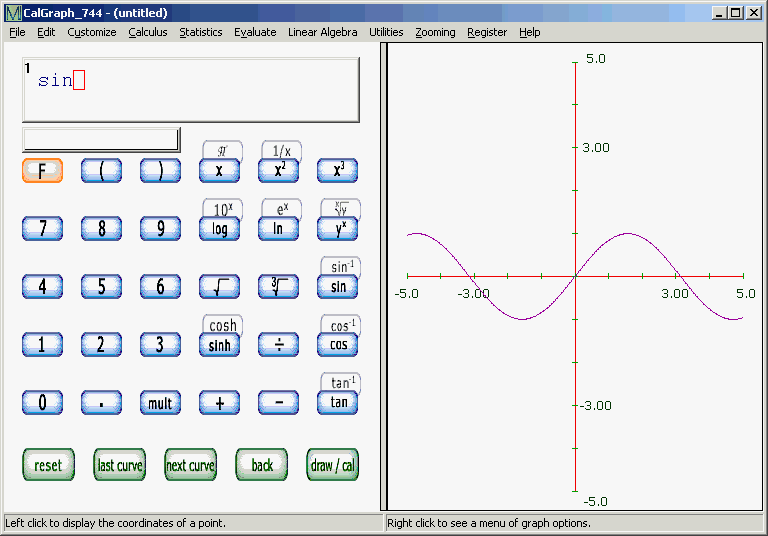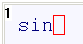# Relaxed Syntax

You can be quite casual about how you enter simple formulas.If CalGraph can figure out what you meant, it plots it for you. Here CalGraph decided thatin the box meant plot the graph of y = sin(x).

Normally you enter  sinx or  sin(x) in the box.

If the expression is not so simple, be careful about how you write the formulas. Use brackets to ensure things are calculated in the right order.